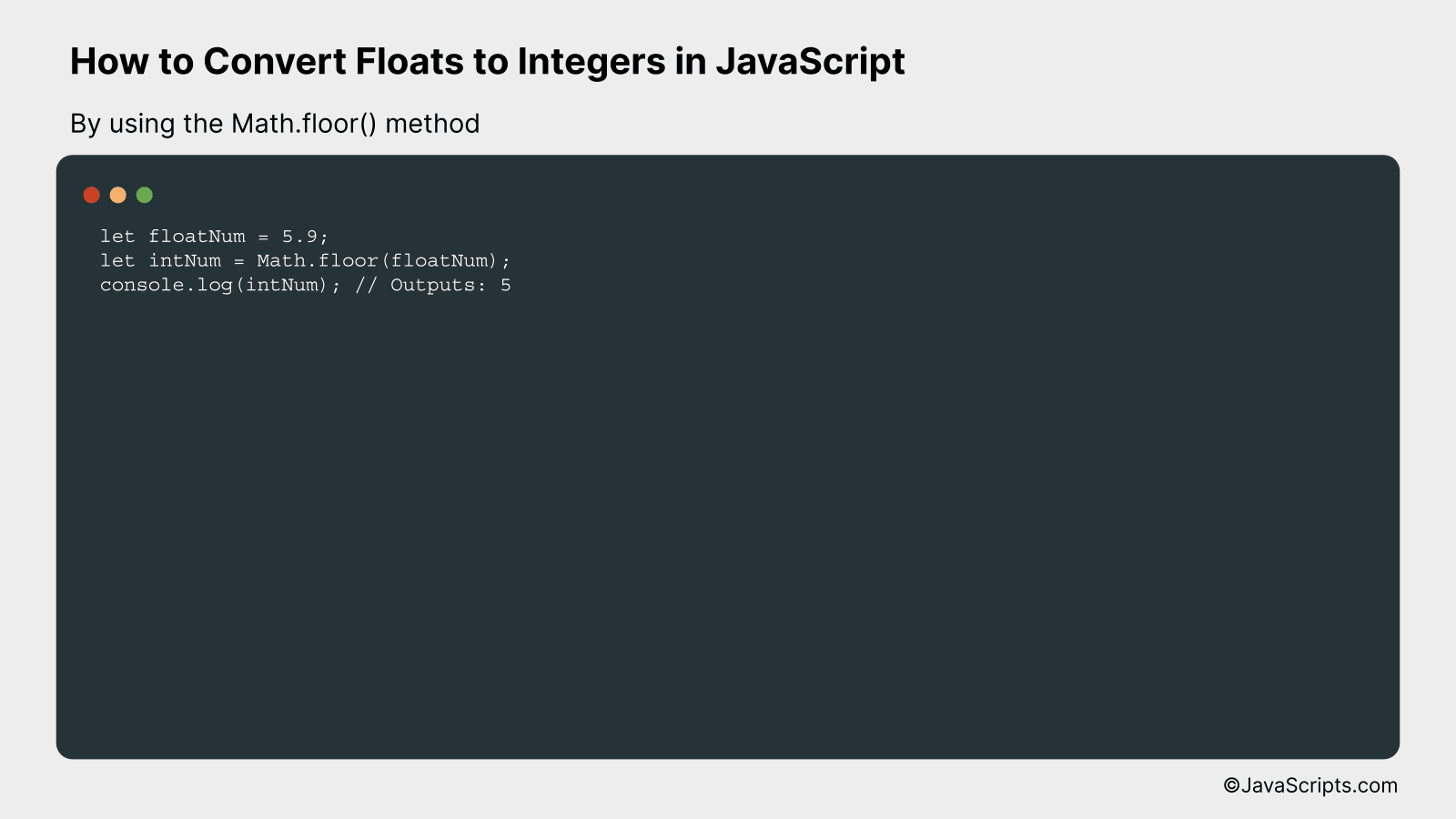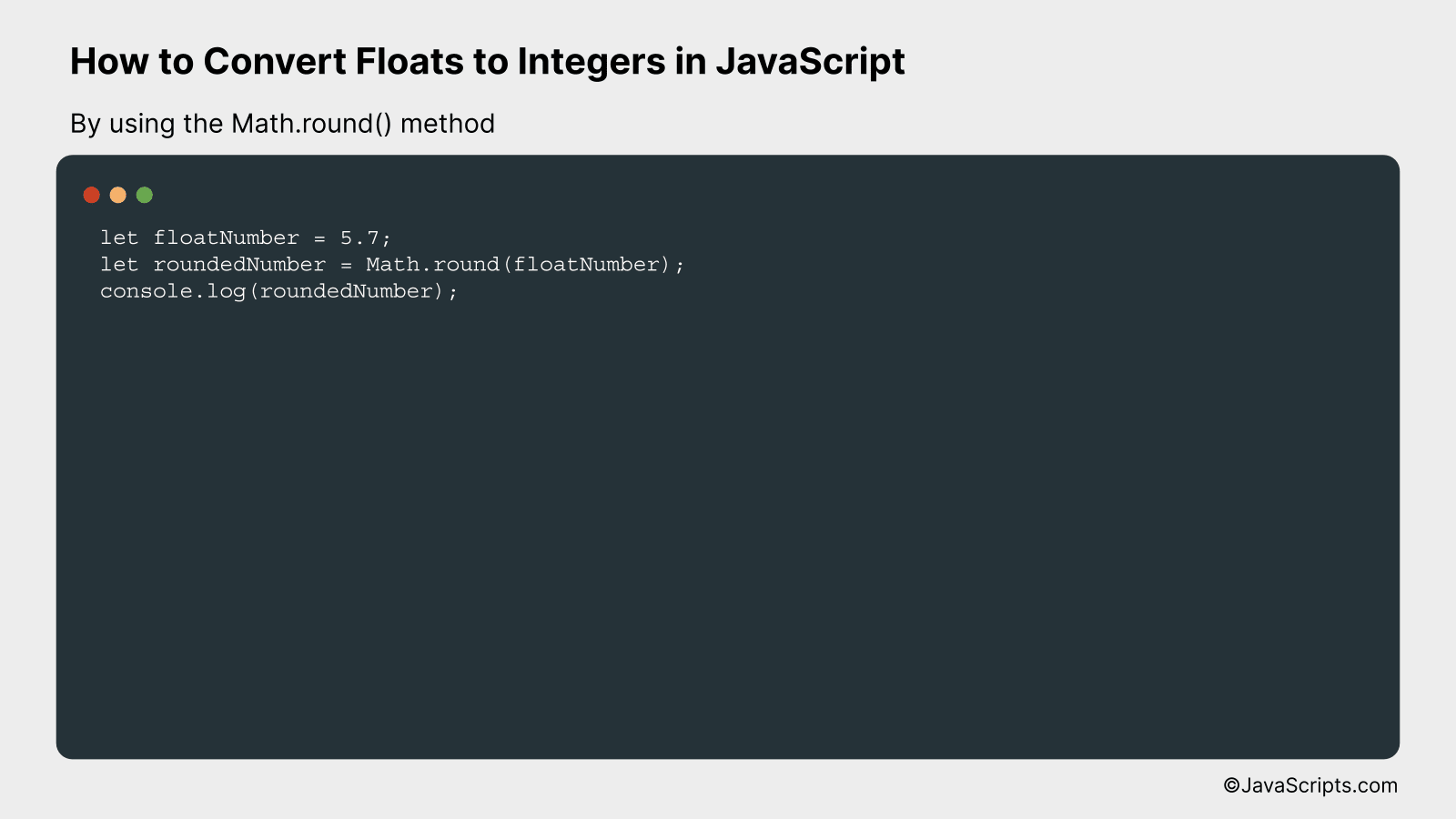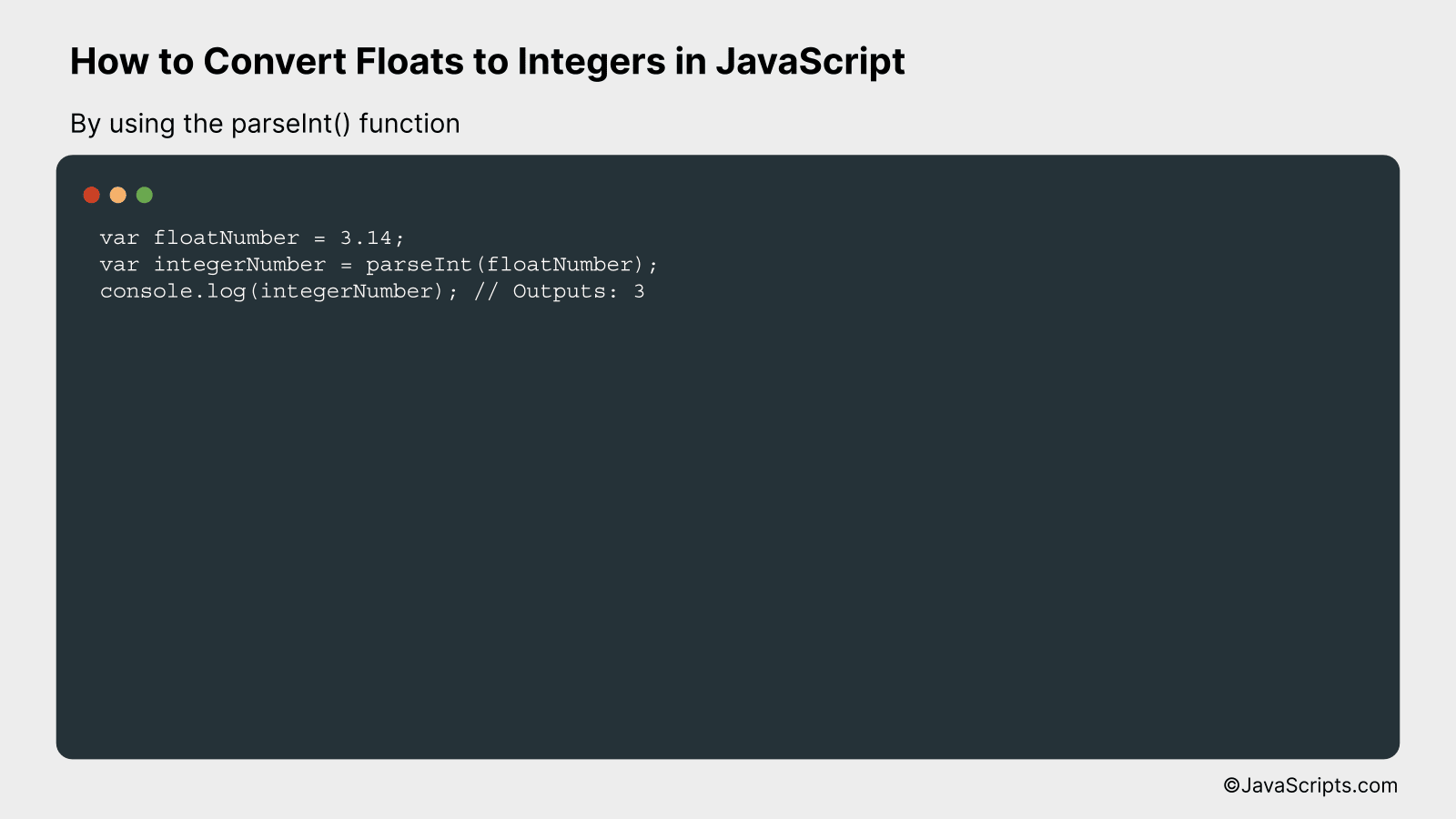# How to Convert Floats to Integers in JavaScript

JavaScript, a powerful and dynamic programming language, allows us to work with different data types. Among these, integers and floating-point numbers, or “floats,” are widely used. Converting floats into integers might seem challenging, but it’s simpler than you think.

You need not be an experienced coder to carry out this conversion. With a basic understanding of JavaScript, you can quickly transform floats into integers. This skill can be a handy tool in your coding toolkit, enabling you to handle numerical data more efficiently.

In this post, we will be looking at the following 3 ways to convert floats to integers in JavaScript:

• By using the Math.floor() method
• By using the Math.round() method
• By using the parseInt() function

Let’s explore each…

## #1 – By using the Math.floor() methodThe Math.floor() method in JavaScript is used to round down a number to the nearest integer. It will convert a floating-point number to an integer by cutting off the decimal portion, effectively ‘flooring’ the value. For example, if you have 5.9 and you use Math.floor(), it will give you 5.

``````
let floatNum = 5.9;
let intNum = Math.floor(floatNum);
console.log(intNum); // Outputs: 5
```
```

### How it works

The Math.floor() method works by removing the fractional part of the given number, leaving the integer part only. It essentially rounds down the number.

• Step 1: Declare a variable ‘floatNum’ and initialize it with a floating-point number, for example, 5.9.
• Step 2: Declare another variable ‘intNum’ and assign it the value of ‘floatNum’ after passing it to the Math.floor() function. This will round down ‘floatNum’ to the nearest integer.
• Step 3: Use console.log(intNum) to print the result. The console will display ‘5’, which is the integer part of the original floating-point number.

## #2 – By using the Math.round() methodThe Math.round() method in JavaScript rounds a floating-point number to the nearest integer. If the fractional part of the number is .5 or more, it rounds up, otherwise it rounds down. Here’s an example:

``````
let floatNumber = 5.7;
let roundedNumber = Math.round(floatNumber);
console.log(roundedNumber);
``````

### How it works

The Math.round() method works by rounding a floating-point number (a number with decimal points) to its nearest integer. It uses a simple rule: if the fractional part of the number is 0.5 or more, it rounds up, otherwise it rounds down.

• Step 1: Define a floating-point number. In the example above, we defined `floatNumber` and set it equal to 5.7.
• Step 2: Use the Math.round() method to round the floating number. We assigned the result to a new variable, `roundedNumber`.
• Step 3: Print the result to the console. In this case, `console.log(roundedNumber)` will output `6` because 5.7 is rounded up to 6.

## #3 – By using the parseInt() functionThe parseInt() function in JavaScript is used to convert a float (or any other number or string) to an integer by dropping the decimal part. For instance, if we pass 3.14 to the parseInt() function, it will return 3.

``````
var floatNumber = 3.14;
var integerNumber = parseInt(floatNumber);
console.log(integerNumber); // Outputs: 3
``````

### How it works

The parseInt() function basically takes a number or a string as an argument and converts it into an integer. If the provided value is a float, it simply disregards everything after the decimal point.

• The variable ‘floatNumber’ is initialized with a float value, here it is 3.14.
• The ‘parseInt()’ function is then called with ‘floatNumber’ as the argument. This function converts the float to an integer by eliminating the decimal part.
• The result is stored in the ‘integerNumber’ variable.
• Finally, ‘console.log(integerNumber)’ is used to print the result, which in this case will be ‘3’.

Related:

In conclusion, converting floats to integers in JavaScript is a simple but useful process. It helps in eliminating decimal points that might be unnecessary for certain calculations or display purposes.

There are various methods, like Math.floor(), Math.ceil(), Math.round(), and the bitwise OR operator. Remember to choose the one that best fits your specific needs. Happy coding!